# Contents

#library(TDbasedUFEadv)

# 1 Introduction

Since TDbasedUFEadv is an advanced package from TDbasedUFE (Taguchi 2023), please master the contents in TDbasedUFE prior to the trial of this package.

## 1.1 Motivations

Since the publication of the book (Taguchi 2020) describing the methodology, I have published numerous papers using this method. In spite of that, very limited number of researcher used this method, possibly because of unfamiliarity with the mathematical concepts used in this methodology, tensors. Thus I decided to develop the packages by which users can use the methods without detailed knowledge about the tensor.

# 2 Integrated analysis of two omics data sets

## 2.1 When features are shared.

### 2.1.1 Full tensor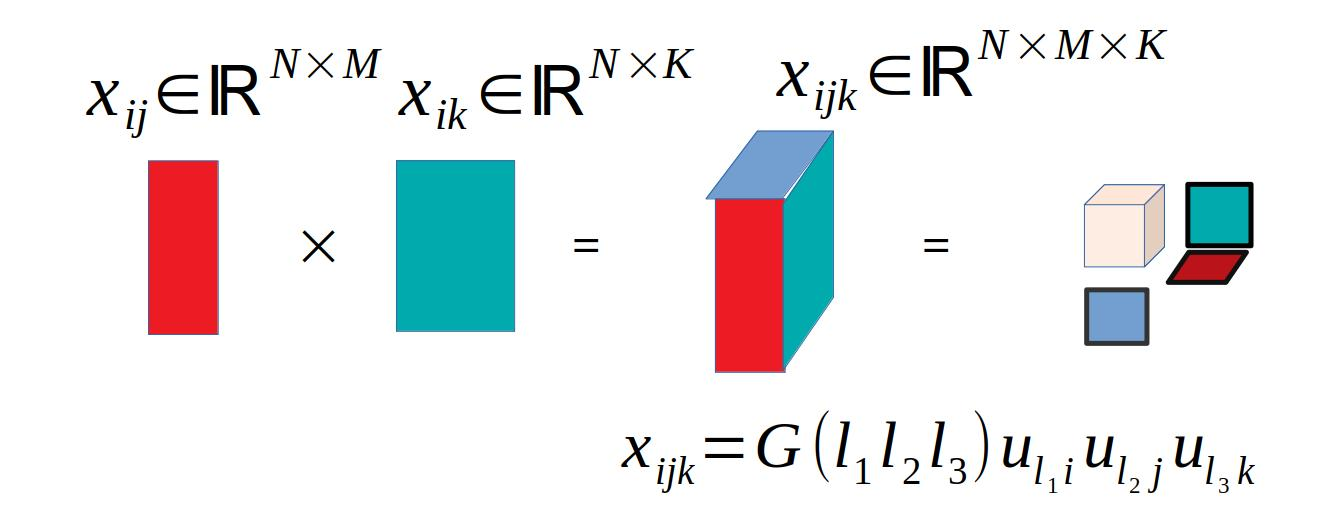Tensor decomposition towards tensor generated from two matrices

Suppose we have two omics profiles $x_{ij} \in \mathbb{R}^{N \times M} \\ x_{ik} \in \mathbb{R}^{N \times K}$ that represent values of $$i$$th feature of $$j$$th and $$k$$th objects, respectively (i.r., these two profiles share the features). In this case, we generate a tensor, $$x_{ijk}$$, by the product of two profiles as $x_{ijk} = x_{ij}x_{ik} \in \mathbb{R}^{N \times M \times K}$

and HOSVD was applied to $$x_{ijk}$$ to get $x_{ijk} = \sum_{\ell_1} \sum_{\ell_2} \sum_{ell_3} G(\ell_1 \ell_2 \ell_3) u_{\ell_1 i} u_{\ell_2 j} u_{\ell_3 k}$ After that we can follow the standard procedure to select features $$i$$s associated with the desired properties represented by the selected singular value vectors, $$u_{\ell_2 j}$$ and $$u_{\ell_3 k}$$, attributed to objects, $$j$$s and $$k$$s.

### 2.1.2 Matrix generated by partial summation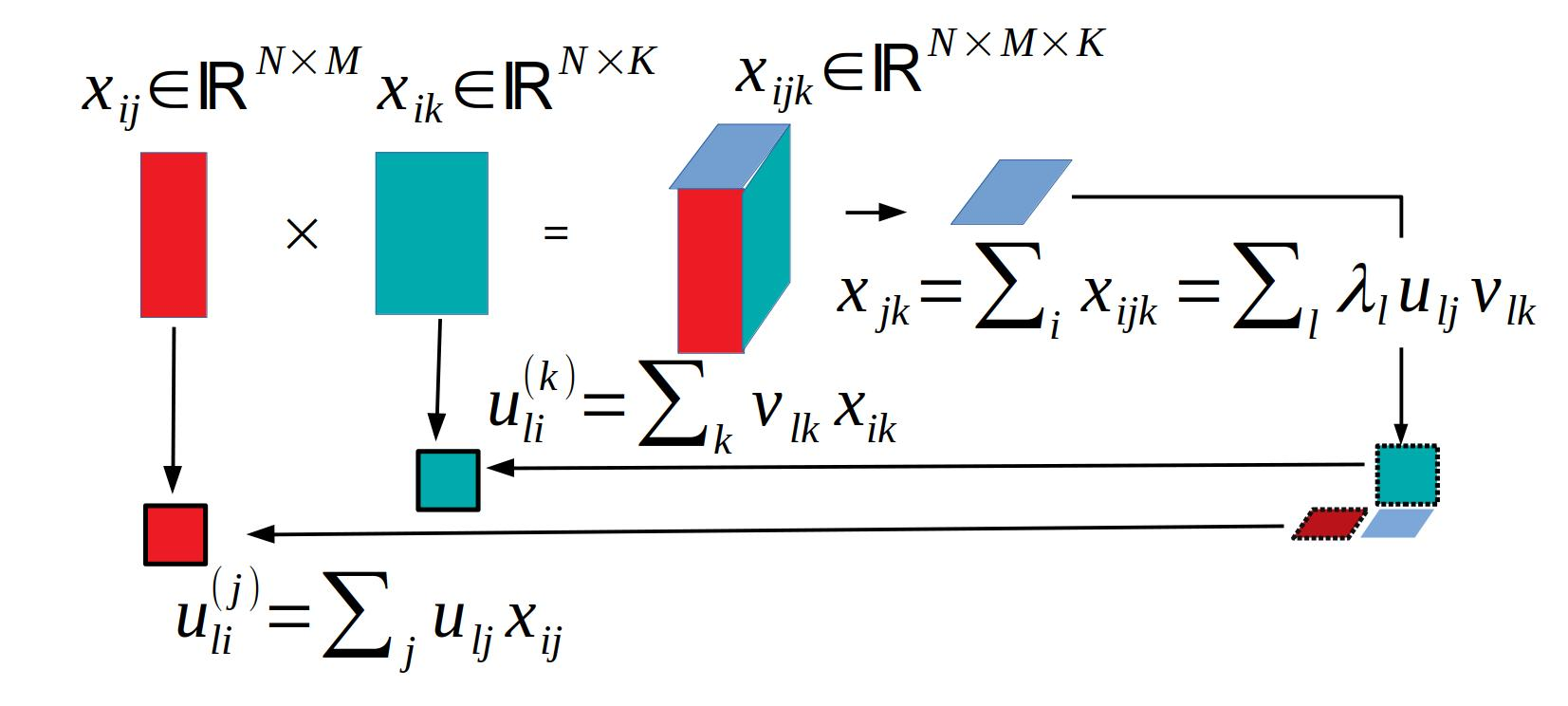After partial summation of tensor

In the above, we dealt with full tensor. It is often difficult to treat full tensor, since it is as large as $$N \times M times K$$. In this case, we can take the alternative approach. In order that we define reduced matrix with taking partial summation $x_{jk} = \sum_i x_{ijk}$ and apply SVD to $$x_{jk}$$ as $x_{jk} = \sum_\ell \lambda_\ell u_{\ell j} v_{\ell k}$ and singular value vectors attributed to samples as $u^{(j)}_{\ell i} = \sum_j u_{\ell j} x_{ij} \\ u^{(k)}_{\ell i} = \sum_k v_{\ell k} x_{ik}$ In this case, singular value vectors are attributed separately to features associated with objects $$j$$ and $$k$$, respectively.

The feature selection can be done using these singular value vectors associated with selected singular value vectors attributed to samples, $$j$$ and $$k$$.

## 2.2 When samples are shared.

### 2.2.1 Full tensor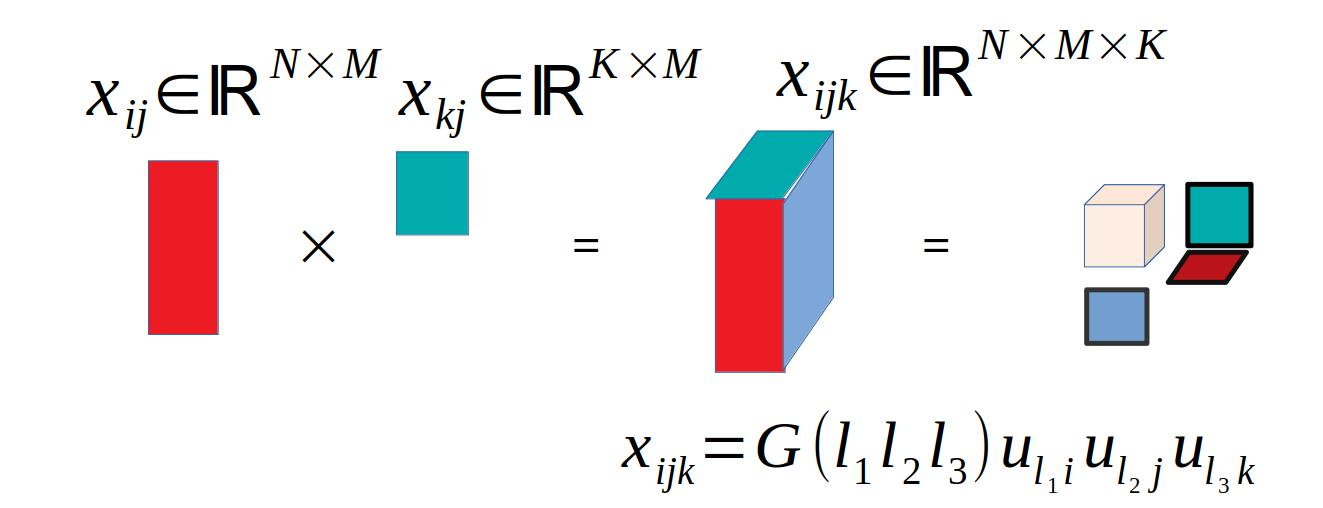Tensor decomposition towards tensor generated from two matrices

In the case where not features but samples are shared between two omics data, we can do something similar. $x_{ij} \in \mathbb{R}^{N \times M} \\ x_{kj} \in \mathbb{R}^{K \times M}$

In this case, we generate a tensor, $$x_{ijk}$$, by the product of two profiles as $x_{ijk} = x_{ij}x_{kj} \in \mathbb{R}^{N \times M \times K}$

and HOSVD was applied to $$x_{ijk}$$ to get $x_{ijk} = \sum_{\ell_1} \sum_{\ell_2} \sum_{ell_3} G(\ell_1 \ell_2 \ell_3) u_{\ell_1 i} u_{\ell_2 j} u_{\ell_3 k}$ After that we can follow the standard procedure to select features $$i$$s and $$k$$s associated with the desired properties represented by the selected singular value vectors, $$u_{\ell_2 j}$$, attributed to objects, $$j$$s.

### 2.2.2 Matrix generated from partial summation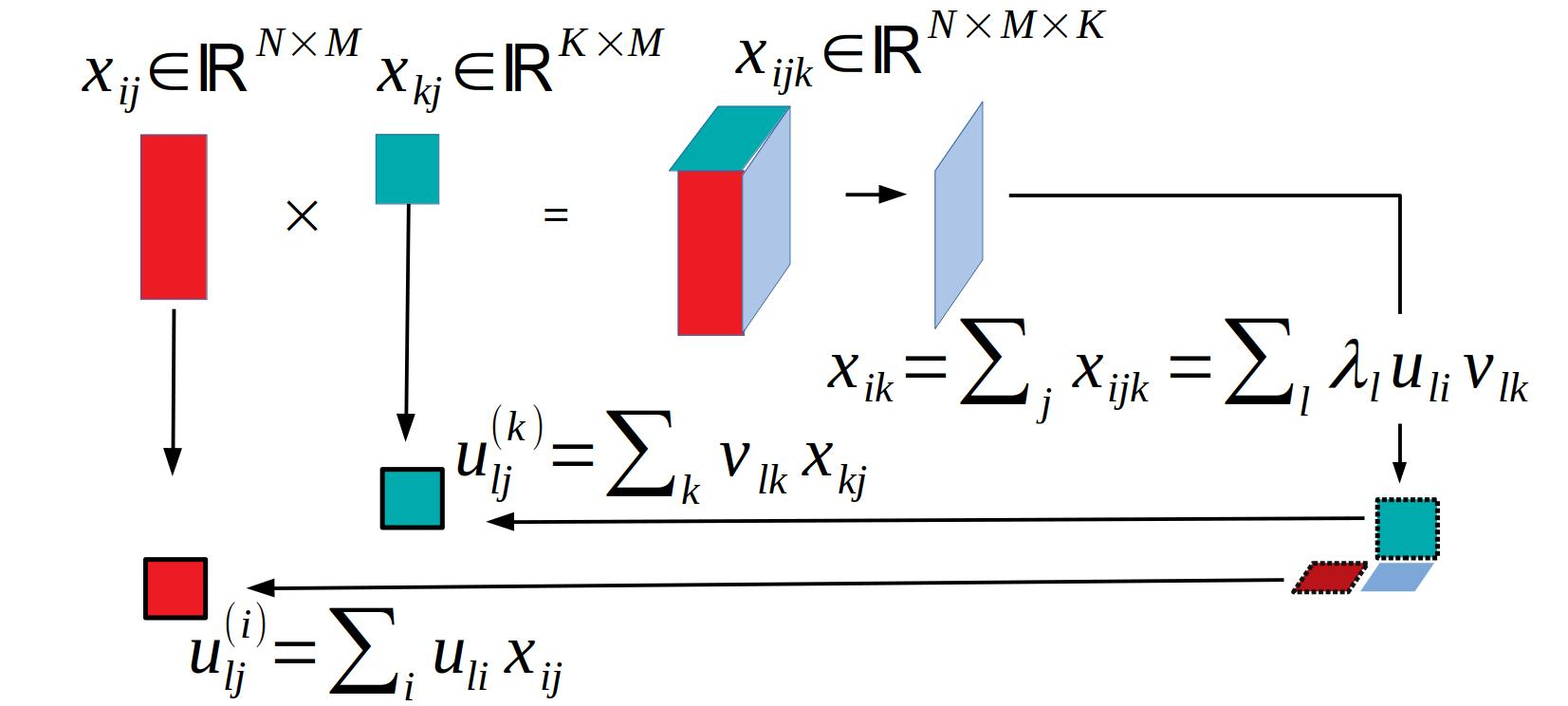After partial summation of tensor

In the above, we dealt with full tensor. It is often difficult to treat full tensor, since it is as large as $$N \times M times K$$. In this case, we can take the alternative approach. In order that we define reduced matrix with taking partial summation $x_{ik} = \sum_j x_{ijk}$ and apply SVD to $$x_{jk}$$ as $x_{ik} = \sum_\ell \lambda_\ell u_{\ell i} v_{\ell k}$ $$i$$s and $$k$$s are selected with $$u_{\ell i}$$ and $$v_{\ell k}$$, respectively. Singular value vectors attributed to samples can be computed as $u^{(i)}_{\ell j} = \sum_i u_{\ell i} x_{ij} \\ u^{(k)}_{\ell j} = \sum_k v_{\ell k} x_{kj}$

# 3 Integrated analysis using projection

Here we would like to propose an alternative strategy to integrate multiple tensors using projection with SVD.

## 3.1 When samples are shared.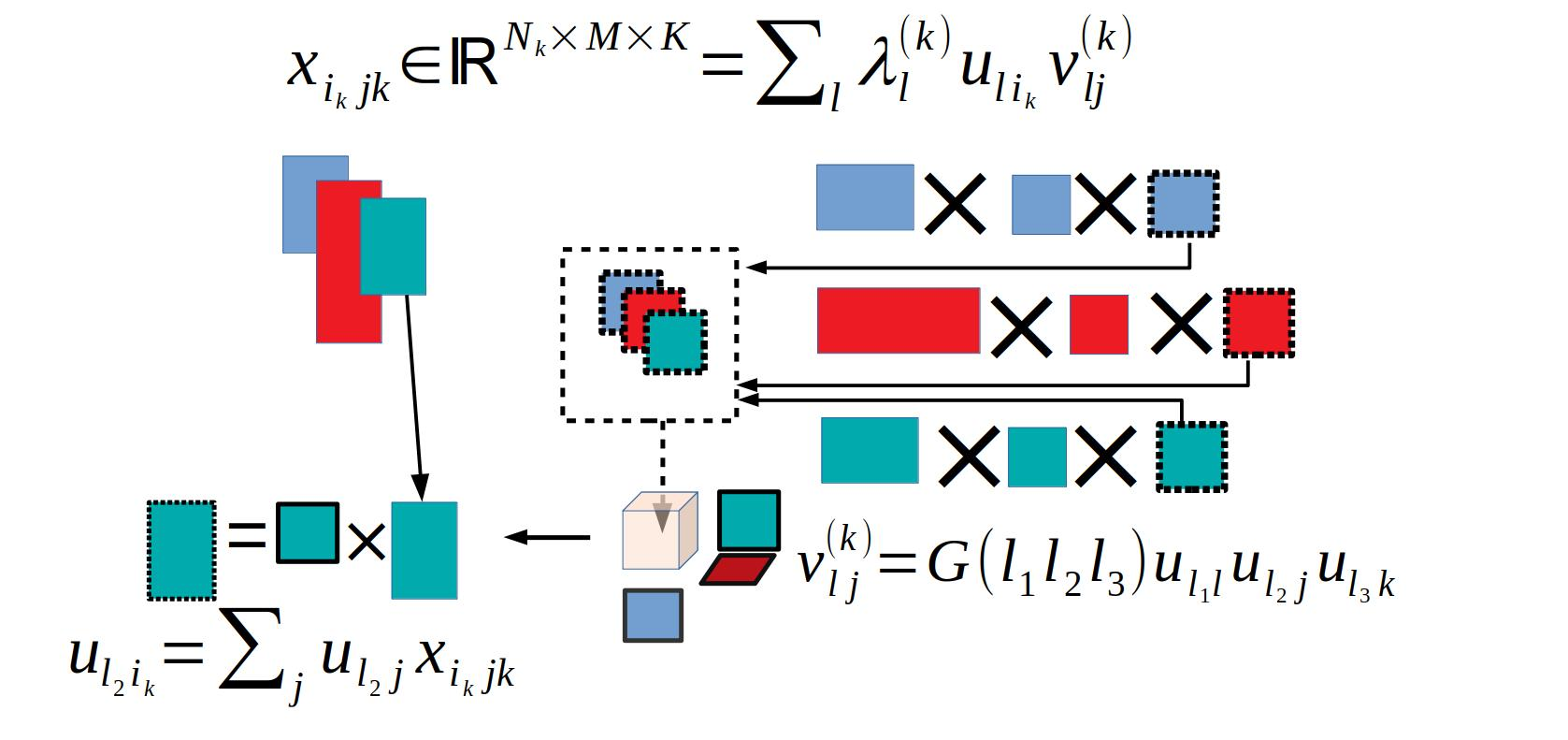Projection when samples are shared

Suppose we have multiomics data as $x_{ijk} \in \mathbb{R}^{N_k \times M \times K}$ for $$i$$th feature of $$j$$th sample at $$k$$th omics data.

In order to bundle them into a tensor, we applied SVD to them as $x_{ijk} = \sum_{\ell} \lambda^{(k)}_\ell u_{\ell i_k} v^{(k)}_{\ell j}$

Then apply HOSVD to $$v^{(k)}_{\ell j}$$ as $v^{(k)}_{\ell j} = \sum_{\ell_1} \sum_{\ell_2} \sum_{\ell_3} G(\ell_1 \ell_2 \ell_3) u_{\ell_1 \ell } u_{\ell_2 j} u_{\ell_3 k}$ After identifying the $$u_{\ell_2j}$$ and $$u_{\ell_3 k}$$ of interest, we can compute $$u_{\ell i_k}$$ as $u_{\ell_2 i_k} = \sum_j u_{\ell_2 j} x_{i_k j k}$ Then $$i_k$$ can be selected as usual.